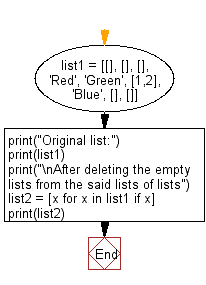﻿ Python: Remove empty lists from a given list of lists - w3resource# Python: Remove empty lists from a given list of lists

## Python List: Exercise - 141 with Solution

Write a Python program to remove empty lists from a given list of lists.

Sample Solution:

Python Code:

``````list1 = [[], [], [], 'Red', 'Green', [1,2], 'Blue', [], []]
print("Original list:")
print(list1)
print("\nAfter deleting the empty lists from the said lists of lists")
list2 = [x for x in list1 if x]
print(list2)
```
```

Sample Output:

```Original list:
[[], [], [], 'Red', 'Green', [1, 2], 'Blue', [], []]

After deleting the empty lists from the said lists of lists
['Red', 'Green', [1, 2], 'Blue']
```

Flowchart:## Visualize Python code execution:

The following tool visualize what the computer is doing step-by-step as it executes the said program:

Python Code Editor:

Have another way to solve this solution? Contribute your code (and comments) through Disqus.

What is the difficulty level of this exercise?

Test your Python skills with w3resource's quiz

﻿

## Python: Tips of the Day

Floor Division:

When we speak of division we normally mean (/) float division operator, this will give a precise result in float format with decimals.

For a rounded integer result there is (//) floor division operator in Python. Floor division will only give integer results that are round numbers.

```print(1000 // 300)
print(1000 / 300)```

Output:

```3
3.3333333333333335```